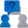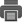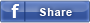• Enquiry

# THOMSON TUBE FOR CALCULATING CHARGE / MASS RATIO OF THE ELECTRON

CAT NO: DIDACTICNPS0016

### Description & Specification:

THOMSON TUBE FOR CALCULATING CHARGE / MASS RATIO OF THE ELECTRON Thomson tube enables to measure the charge/mass ratio of electrons..
Thomson tube is a tube under vacuum; a scale is printed sideways on a slab of semi-transparent mica. This slab is kept in its position by two metallic plates that can generate an electric field E.
Two Helmholtz coils, kept in their position by a universal
support, can generate a magnetic field B that forms specific
angles with the electric field.
A fluorescent substance available on the opposite side of
mica slab will show the parabolic path of the electronic beam
in an electric field, and the circular path of the same ray in a
magnetic field.
A hot filament will generate a beam of electrons. This beam
is accelerated through a magnetic and electric field, arranged
at prefixed angles between each other, and it follows a
perpendicular path to the electric field. Using the formula of
kinetic energy and the Lorenz equation that describes the
force on a charged particle in a magnetic and electric field, it is
possible to calculate the charge to mass ratio of the electron.

COMPONENTS
• universal support
• Helmholtz coils
• Thomson tube for calculating e/m ratio
• power supply units

Supplied with THEORETICAL – EXPERIMENTAL HANDBOOKAdd to Wish List